# ohms law...how much should i round up when the answer is a long fraction?

#### kapijapi1

Joined Mar 29, 2020
1
So i got to this part about series-parallel circuits from the education tab and im a bit confused.

first the author calculates the parallel resistor's resistance and combines them to get a single resistor to make it a series circuit. the total resistance for the first one is 71.42857142857143 but he just shortens it to 71.429 then later on when he finds the voltage drops the first one is 8.6275591343733427 but he writes it as 8.6275 instead.

my question is why does he sometimes write it with 2 numbers after the decimal point and sometimes more. It might seem like a dumb question but when i calculated the current for the first parallel circuit with 8.6275 it came as 34.51mA but when i did it with shorter number 8.63 the answer came to be 35.52mA. if i were to use the shorter number then the result would of been different

So my question is when should i round down the number to 2 decimal places and when should i round it to more than 2 decimal places?

i have included a link at the bottom to the text in case you would want to see for yourself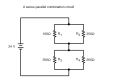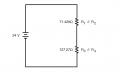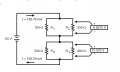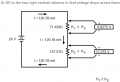#### MrChips

Joined Oct 2, 2009
25,932
This is more a question about precision and significant figures.
You need to understand what is important.

Consider that 100 years ago commercially available resistors had a tolerance of ±20%. That meant that if you bought a 100Ω resistor you could expect the resistance to be somewhere between 80 and 120Ω

Fifty years later 10% resistors became readily available. Today, many electronic circuits will work well with 5% resistors.
If you want to be obsessed with accuracy you can stock your electronics parts bins with 1% resistors.

So let us put this into its proper perspective. If your calculations give you an answer of 100.45678912345Ω
how many decimal places would you need to be within ±1% accuracy?

The answer is 0 decimal places. An answer of 100 is good to ±1%.

So the real answer to your question is not how many decimal places you need to write down but what percentage error is adequate. If you want to avoid propagating rounding errors then use better than ±1%

So for a result such as 8.6275591343733427 a proper answer is 8.63, in other words three significant figures.
An answer of 765432.01234 would become 765000, i.e. three significant figures (not decimal places).

btw, this does not apply only to Ohm's Law and electronics. It applies to all fields of engineering. Some calculations might demand 4, 5, or 6 significant figures. It most cases 3 significant figures are good enough.

#### wayneh

Joined Sep 9, 2010
17,167
You'll note that the author used the same number of significant figures in both cases, not the same number of digits after the decimal. Using significant figures consistently is justified as @MrChips described - an error in the last digit corresponds roughly to a given percentage error. There's no justification for using a certain number of digits after the decimal, since the decimal placement is due to an arbitrary choice of units, ohms versus milliohms or megohms for instance.

#### crutschow

Joined Mar 14, 2008
29,521
Note that when you doing the calculations (at least with a computer or calculator) you want to use all the digits as you continue the calculation, to minimize round-off error in the final result.
But when the result is displayed it is often not needed to show more that about 0.1% to 0.01% precision for engineering use.

#### dl324

Joined Mar 30, 2015
14,332
my question is why does he sometimes write it with 2 numbers after the decimal point and sometimes more.
No rhyme nor reason.

Just use common sense to determine how many significant digits to keep and when to round up or down.

If you want the numbers in the circuit you showed to "add" up, do all of the calculations in one step.

If you call the node between the two parallel sets of resistors A,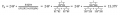#### wayneh

Joined Sep 9, 2010
17,167
I’ve used lab and engineering instruments throughout 4 decades. The degree of accuracy and precision you require has cost.

Three significant figures is relatively easy and cheap. A typical home thermometer can be read to 3 sig figs.

Four significant figures is a lot tougher. Ten times? Probably more. That’s like measuring your body temperature to 98.60. In most fields, like biology or weather, that level isn’t even meaningful. You’d be laughed at for publishing numbers like that. A false precision. It’s achievable in electronics, though, and in the physical sciences. A bathroom scale can do it.

Five significant figures is almost unobtainable without expensive equipment and extreme care. A good analytical balance can do it but I can think of few others.

Getting six figures with even an analytical balance requires an isolated, vibration-proof room with custom ventilation and probably other precautions I don’t recall.

But as suggested, calculations can and should be done with the full precision possible. Just don’t report your results to 12 figures.

#### DarthVolta

Joined Jan 27, 2015
521
When using sig. fig's, what about moving across orders of magnitude ? Like rounding AC base current's of nano-amp's in 1 calculation, and then deciding what to round some resistance to, that is on the order of 10k or 100k. I'm sure there's a good math reason for something, but I don't remember what it is

#### wayneh

Joined Sep 9, 2010
17,167
When using sig. fig's, what about moving across orders of magnitude ? Like rounding AC base current's of nano-amp's in 1 calculation, and then deciding what to round some resistance to, that is on the order of 10k or 100k. I'm sure there's a good math reason for something, but I don't remember what it is
The exponents don’t matter, only the significant figures. For instance a probability could be called 1 or it could be 100%. That’s the same thing and one SF either way. Or 0.99 is the same as 99%. That’s two SF. And so on.

#### SamR

Joined Mar 19, 2019
4,132
Note that when you doing the calculations (at least with a computer or calculator) you want to use all the digits as you continue the calculation, to minimize round-off error in the final result.
In addition, using a calculator, I keep it in engineering notation and float. When I get to the end of the calculation in M, k, m, u, n, p, or any other unit a decimal with 2-3 places after for the solution. Keeping all the units the calculator provides during the multistep calculations shaves off the accumulated error from continual rounding during each step. That is not the "Book" method but what I have used that works for me. YMMV I hated constant rounding with a slide rule. Call me an anal-retentive perfectionist if you wish.

#### WBahn

Joined Mar 31, 2012
26,398
A common -- and almost always sufficient -- result is to report results to 3 sig figs. In most engineering situations, 2 is really all that is necessary (there are most certainly exceptions). To minimize cumulative round off errors if you can't let the calculator/computer track them out as far as it can, use at least one and preferably two additional sig figs on intermediate results. This works well almost all the time, but again there are exceptions.

You would be well advised to read up on accuracy, precision, and propagation of errors.